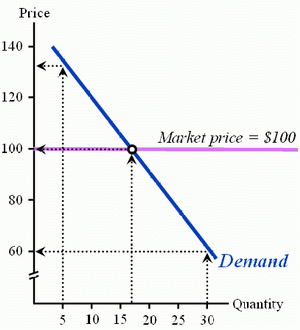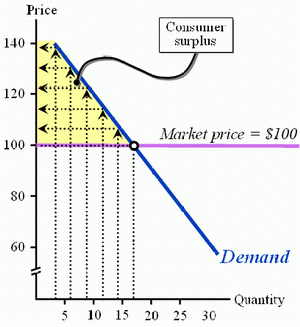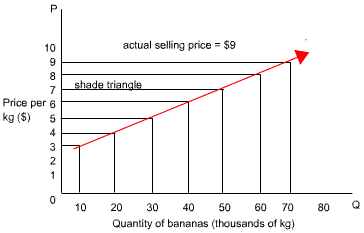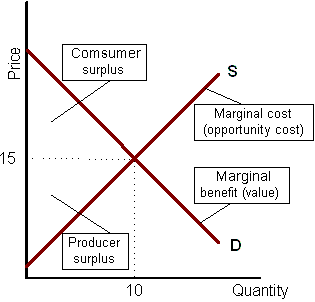Subject 5. Consumer Surplus, Producer Surplus, and Total Surplus
#cfa #cfa-level-1 #economics #has-images #microeconomics #reading-13-demand-and-supply-analysis-introduction
Consumer Surplus

Consumer surplus is the area below the demand curve but above the actual price paid. It is the difference between the amount consumers are willing to pay and the amount they have to pay for a good.

Consider the market for a good.• If the market price is $100, then the 30th unit will not sell because those who demand it are only willing to pay$60 for the good.
• At $100, the 17th unit will sell because those who demand it are willing to pay up to$100 for the good.
• At $100, the 5th unit will sell because those who demand it are willing to pay up to$133 for the good.• For all those goods under 17 units, people are willing to pay more than $100. • The area represented by the distance above the actual price paid and below the demand curve is called consumer surplus. • This area represents the net gains to buyers from market exchange. Lower market prices increase the amount of consumer surplus in the market. Producer Surplus Producer surplus is the difference between the minimum supply price, represented by the supply curve, and the actual sales price. • It is measured by the area below the price and above the supply curve, up to the quantity sold. • It accrues to owners of firms and resource suppliers. ExampleThe actual selling price of bananas is$9 per kg.

Now imagine that there are only 10,000 kg bananas being supplied at the moment. The marginal cost per kg is only $3 but the selling price is$9. So there is an additional $6 per kg being raised. This is the producer surplus per kg. The total producer surplus when 10,000 kg are produced is thus$60,000.

Due to the surplus, more producers enter the market and another 10,000 kg are produced, so there are now 20,000 kg of bananas on the market. The marginal costs of producing this additional 10,000 kg have risen to \$4.

Producer surplus is not the same as profit. Remember that profit is the return that accrues to owners of a firm and is the difference between sales revenue and total (not marginal) costs of production.

Total Surplus

The following figure shows that a competitive market creates an efficient allocation of resources at equilibrium.• In equilibrium, the quantity demanded equals the quantity supplied.
• At the equilibrium quantity, marginal benefit equals marginal cost, so the quantity is the efficient quantity.
• The sum of consumer and producer surplus is maximized at this efficient level of output. It is here that all potential gains from production and exchange are realized.
If you want to change selection, open original toplevel document below and click on "Move attachment"